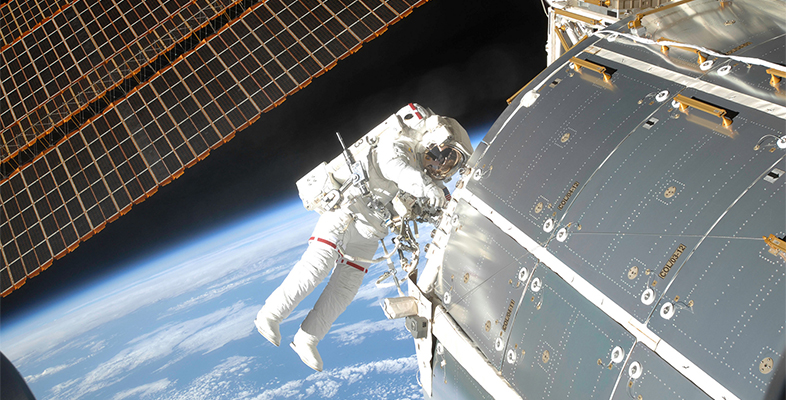Science, Maths & Technology

### Become an OU studentMicrogravity: living on the International Space Station

Start this free course now. Just create an account and sign in. Enrol and complete the course for a free statement of participation or digital badge if available.

# 1 How much does the ISS cost?

Recall Video 2 [Tip: hold Ctrl and click a link to open it in a new tab. (Hide tip)] in Week 4 which stated that the ISS alone had cost between US$140 and 160 billion! Figure 1 shows the cost history of the ISS for the period between the financial years (FY) 1995 and 2002. Note that 2002 has two cost elements – ‘assembly complete’ and ‘core complete’. Please ignore the last column for ‘core complete’ as these values are taken forward into 2004 and 2006.Figure 1 Cost history of the ISS. Now use Figure 1 to complete Activity 1. ## Activity 1 The cost history of the ISS Timing: Allow approximately 10 minutes Choose the correct answer to each of the following questions. 1. Which year was the most expensive? a. 1995 b. 1999 c. 2000 d. 2001 e. 2002 The correct answer is e. 2. What was the total cost in billion dollars between 1995 and 2002? a. US$145.2 billion

b.

US$169.1 billion c. US$185.2 billion

d.

US$205.2 billion e. US$295.2 billion

3. What was the average cost between 1995 and 2002? (Hint: divide the total cost in question 2 by the number of years.)

a.

US$19.14 billion per year b. US$20.14 billion per year

c.

US$21.14 billion per year d. US$22.14 billion per year

e.

US\$23.14 billion per year